﻿ Statistical Thinking in Problem SolvingPublications are Open
Access in this journal
Article Versions
Export Article
• Normal Style
• MLA Style
• APA Style
• Chicago Style
Research Article
Open Access Peer-reviewed

### Statistical Thinking in Problem Solving

Michael Gr. Voskoglou , Evangelos Athanassopoulos
American Journal of Educational Research. 2020, 8(10), 754-761. DOI: 10.12691/education-8-10-3
Received September 02, 2020; Revised October 04, 2020; Accepted October 13, 2020

### Abstract

This article studies the role of Statistical Thinking in Problem Solving., where the problem is considered with its wide meaning (not mathematical problems only). Particular emphasis is given to Bayesian Reasoning, whose importance in everyday life and science applications has been only recently fully recognized. Critical and Computational Thinking, the other two main modes of thinking used in Problem Solving, are also discussed and examples are presented illustrating our conclusions.

### 1. Introduction

The importance of Problem Solving (PS) has been realized for such a long time that in a direct or indirect way affects our daily lives for centuries. Volumes of research have been written about PS and attempts have been made by many educators, psychologists, philosophers and other special scientists to make it accessible to all in various degrees. However, even graduates have nowadays difficulty in solving real life problems. Somehow, they cannot apply theory into practice, or theorize/reflect on practice. In fact, it is the human mind at the end that has to be applied in a problematic situation and solve the problem.

PS is a complex phenomenon and no wonder there is no unique definition about it. The following definitions, however, encompass most of the existing ones: According to Polya 1, the pioneer in mathematical PS, “solving a problem means finding a way out of a difficulty, a way around an obstacle, attaining an aim that was not immediately understandable.”

For Schoenfeld 2 “a ‘problem’ is only a problem, if you don’t know how to go about solving it. A ‘problem’ that has no surprises in store, and can be solved comfortably by routine or familiar procedures (no matter how difficult!) it is not actually a problem, it is an exercise”.

Green and Gilhooly 3 state that PS is an activity that draws together the different components of cognition. Therefore, the kind of the problem dictates the type of cognitive skill necessary to solve it: Linguistic skills are used to read about a certain problem and debate about it, memory skills to recall prior knowledge for solving it and so on. Depending on the knowledge and thinking skills possessed by a problem solver, what could be a problem for one might not be a problem for somebody else.

Perhaps Martinez’s 4 definition carries the modern message about the process of solving problems: “PS can be defined simply as the pursuit of a goal when the path to that goal is uncertain. In other words, it’s what you do when you don’t know what you’re doing”.

In this work, where the problem is considered with its wide meaning (not mathematical problems only), PS is understood to be the activity that makes use of cognitive and physical means to overcome an obstacle (problem) and develop a better idea of the world that surrounds us.

The capacity to solve problems is directly related to the knowledge stored in an individual’s mind, and knowledge is a product of thinking. But thinking can vary from a very simple and mundane thought to a very sophisticated and complex one. The nature of the problem dictates the level of thinking. The present paper focuses on studying the importance of statistical thinking (ST) for PS, although the other forms of thinking needed for PS are also discussed. The term ST here is understood to be the type of thinking which is based on the laws of Probability and Statistics.

The rest of the paper is organized as follows: Section 2 looks back to the history of the development of Probability and Statistics. Section 3 discusses the different modes of thinking needed in PS with particular emphasis to ST. Section 4 is devoted to Bayesian Reasoning and the paper closes with the general conclusions presented in Section 5.

### 2. Looking Back to the Development of Probability and Statistics

Probability and Statistics are related areas of mathematics, having however fundamental differences. Probability is a theoretical branch of mathematics which deals with predicting the likelihood of future events. On the contrary, Statistics is an applied branch of mathematics, which tries to make sense of observations in the real world by analyzing the frequency of past events. The distinction between Probability and Statistics could be clarified better by tracing the thoughts of a gambler mathematician during a game with dice. If the gambler is a specialist in probability, he will think that each face of the dice comes up with probability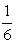. If instead he is a statistician, he will think: “How I know that the dice are not loaded? I keep track how often each number comes up and once I am confident that the dice are fair I’ll decide how to play”. In other words, Probability enables one to predict the consequences of an ideal world, whereas Statistics enables him/her to measure the extent to which our world is ideal.

Probability theory has been developed in response to the humans’ tendency to deal with games of chance. Famous mathematicians of the 17th and 18th century like Fermat, Pascal, Bernoulli, De Moivre and others, put the frames of the corresponding theory by introducing methods for solving problems related to the games of chance. However, the appearance of Probability as an independent branch of mathematics is due to the Laplace’s (1749-1827) book “Theorie Analytique de Probabilite”, which was published in 1812.

Another important step forward was the Bayes’ Rule of calculating the value of conditional probabilities, which first appeared in the work “An Essay towards a Problem in the Doctrine of Chances”, published by Richard Price in 1763, two years after the Bayes’ (1701-1761) death. Laplace, independently from Bayes, pioneered and popularized the conditional probabilities. Due to its great importance for the whole science, the Bayes’ rule will be studied in a separate section of this work. It must be mentioned here, however, that the Bayesian approach used to be for years the reason of a continuous dispute, mainly on philosophical level, between the supporters of it and the supporters of the Laplace’s “classical” approach of probability.

A fundamental difference was, for example, that the Laplace’s supporters believed that the probabilities exist only for events that have not happened yet, whereas, according to the Bayesian approach, this is not a necessary condition. To make it clearer, assume that one throws a weighted coin, covers it with his hand and then bets if the indication was “head” or “letters”. According to the Bayesian approach, the probability of appearance of each indication is 50%, whereas according to the classical approach such a probability does not exist, since the event has already happened! It is only recently that the “opponents” of this cognitive fight have been convinced that the two views could exist together and they are equally useful for the scientific progress.

Looking back to the invention of Statistics one finds the name of John Graunt (1620-1674), who developed early human statistical and census methods that provided a framework for the modern demography. Although a haberdasher by profession, Graunt used his knowledge in mathematics to produce the first life table giving probabilities of survival to each age. He is also considered as one of the first experts in epidemiology, since his famous book “Natural and Political Observations Made Upon the Bills of Mortality” was concerned mostly with public death statistics. The book, who led Graunt to be elected as a fellow of the London Royal Society, ran five editions, the first being in 1662 (Figure 1) and the last after his death in 1676. The success of Graunt’s methods led, around 1700, to the use of Statistics to problems related to the games of chance first and gradually to the whole science as well.

The simultaneous and collaborative use of Probability and Statistics in everyday life and science applications led, among others, to the Von Mises’ (1883-1953) statistical definition of probability, which, in contrast to the Laplace’s classical or mathematical definition, holds even for infinite sample spaces with no equally probable singleton events. The statistical definition, however, assumes that an experiment of chance could be repeated as many times as the observer wishes, which does not happen frequently in practice. The Kolmogorov’s (1903-1987) axiomatic definition came finally to bridge the existing gap by generalizing both the previous two definitions, although it does not provide a unique measure for probability 5.

• Figure 1. The title page of the 1st edition of the Graunt’s book (1662)

Edwin T. Jaynes (1922-1998), Professor of Physics at the University of Washington, was the first who argued that Probability theory could be considered as a multi-valued generalization of the bivalent logic reducing to it in the special case where our hypothesis is either absolutely true or absolutely false 6. Many eminent scientists have been inspired by the ideas of Jaynes, like the expert in Algebraic Geometry David Mumford, who believes that Probability and Statistics are emerging as a better way for building scientific models 7.

Nevertheless, both Probability and Statistics have been developed on the basis of the bivalent logic. As a result, they are tackling effectively only the cases of the existing in real world uncertainty which are due to randomness and not those due to imprecision. In cases of imprecision, the Zadeh’s Fuzzy Logic (FL) comes to bridge the existing gap 8. It is recalled that FL is an infinite-valued on the real interval [0, 1] logic, which is based on the concept of Fuzzy Set (FS) introduced by Zadeh in 1965 9. FL, which has found nowadays important applications to almost all sectors of the human activity, does not contradict the Aristotle’s bivalent logic, but it actually generalizes and completes it. For more details about the history, development and the basics of FS and FL the reader may look at 10, Section 2.

### 3. Modes of Thinking in Problem Solving

3.1. The Aristotle’s Statistical Fallacies

Fallacies are logically false statements which are often considered to be true. A great number of fallacies are known nowadays (e.g. see 11), but the first fallacies appeared in the literature simultaneously to the generation of the Aristotle’s (384-322 BC) bivalent Logic. In the “Sophistical Refutations”, the last of his six works on logic, the great ancient Greek philosopher identified thirteen fallacies and divided them in two categories, the linguistic and non-linguistic ones 12. The amazing thing, however, is that, although Statistics was a completely unknown subject on that time, two of the Aristotle’s fallacies can be characterized in nowadays terms as statistical fallacies! Those fallacies are the unqualified and the hasty generalizations respectively. In the first one a general rule is used to explain a specific case that does not fall under this rule, whereas in the second one it is assumed that something is true in general, because it happens to be true in certain cases.

Assume, for example, that a high school employs 100 in total teachers. Three of them are not good, whereas the other 97 are good teachers. Parent A happens to know only the three not good teachers. Based on it, he concludes that the school is not good and he decides to choose another school for his child. On the contrary, parent B, who knows the 97 good teachers, concludes that the school is good and decides to choose it for his child. In that case, parent A has fallen into the fallacy of hasty generalizations, whereas parent B has fallen into the fallacy of unqualified generalizations. It becomes evident, however, that the gravity of the consequences of those two fallacies is not the same. In fact, the degree of truth of the former one is only 3%, whereas the latter has degree of truth 97%. Therefore, the decision of parent A could jeopardize the future of his child, whereas the decision of parent B could possibly benefit his child.

3.2. Critical Thinking

The cultivation of the statistical literacy is very important, but alone is not enough; it must be combined with critical thinking (CrT). It is recalled that CrT is considered to be a higher mode of thinking by which the individual transcends his subjective self in order to arrive rationally at conclusions substantiated using valid information ( 13, Section 3). Through CrT reasoning skills such as analysis, synthesis and evaluation are combined giving rise to other skills like inferring, estimating, predicting, generalising, problem solving, etc. 14.

Socrates (470-399 BC), the “father” of CrT, in his dialogue with his friend Euthydemus - written by his student Plato in 384 BC, i.e. the year of the Aristotle’s birth - exploited tacitly the Aristotle’s statistical fallacies to give the following example about the importance of CtT for PS in general and decision-making in particular. Socrates asked Euthydemus, if he thinks that cheating is immoral. Of course it is, answered Euthydemus. But what happens, replied Socrates, if your friend wants to commit a suicide and you steal his knife? There is no doubt that you cheat him in that case, but is this immoral?. No, said the embarrassed Euthydemus 15. Here Euthydemus followed the statistical way of thinking, since in most cases cheating is considered to be an immoral action. Socrates, however, with this dialogue, taught him that he must combine it with CrT.

Let us now transfer the dialogue of Socrates with Euthydemus to the previous example with the two parents. Imagine that Socrates (if he was alive on that time) met parent B downtown and asked him: If your child has a particular interest about the lessons taught by the three bad teachers and he is not interested about the lessons taught by the 97 good teachers, is your decision to choose that school right for his future?. After this, parent B became puzzled and he thought that he should reconsider his decision after discussing it with his child.

3.3 Other Examples

Apart from the Aristotle’s, many other statistical fallacies are known today, such as the sampling bias, the data dredging, the survivorship bias, the cherry picking, the gambler’s fallacy, the regression toward the mean, the thought-terminating cliché, etc. 16. Many of those fallacies involve lack of CrT as well.

• Figure 2. The Wason’s four card problem

A characteristic example related to lack of ST and CrT in PS is the Wason’s four card problem: A set of four cards is placed on a table, each of which has a number on the one side and a colored patch on the other side. The visible faces of the cards show 3, 8, red and brown respectively (Figure 2). Which cards must be turned over in order to test the truth of the proposition that if a card shows an even number, then its opposite face is red? Since only the cards with number 8 and the red-colored one could contradict the truth of the given proposition, the correct solution is to turn over those two cards. In the Wason’s study less than 10% of the subjects found the correct solution 17, 18.

The famous Linda’s problem 19, a special case of the conjunction fallacy, is also related to lack of ST: Linda is 31 years old, single, outspoken and very bright. She majored in Philosophy. As a student, she was deeply concerned with issues of discrimination and social justice, and also participated in anti-nuclear demonstrations. The subjects were asked to choose which one among five given alternatives is the most probable about Linda. Two of those alternatives stated that “Linda is a bank teller“ and that “Linda is a bank teller and also is active in the feminist movement”.

The majority of the subjects chose the second statement as more probable than the first one. This violates the law that the probability of a conjunction must be less (or equal) than the probability of its conjuncts. Note, however, that according to other researchers, this “irrationality” could be explained better in other ways, e.g. by using a fuzzy instead of probabilistic model, since the given Linda’s description contains many fuzzy characteristics 20.

3.4. Computational Thinking

Living in a knowledge era and increasing progress in technology, combining knowledge and technology to solve problems is becoming the mode rather than the exception. Creativity and innovation driven by tacit knowledge, CrT driven by logic and ST based on the rules of Probability and Statistics have become the tools for problem thinking and PS. If technology is added as another tool then Computational Thinking (CT), in its broader meaning other than performing computations, is also a prerequisite.

The term CT was first used by S. Papert 28, who is widely known for the development of the Logo software. However, it was brought to the forefront of the computer society by Wing 22, to describe how to think like a computer scientist. Wing described CT as “solving problems, designing systems and understanding human behaviour by drawing on the concepts fundamental to computer science”. Liu and Wang 23 emphasize that CT is a hybrid of other modes of thinking, like abstract thinking, logical thinking, modelling thinking, and constructive thinking. CT frees memory so that the problem solver can concentrate on the essence of the problem 24.

Voskoglou and Buckley 13 developed a model shedding some light to the relationship between CT and CrT in PS, which is graphically represented in Figure 3. In this model, the three components of CrT, CT and existing knowledge act simultaneously on the problem at hand. The model is based on the hypothesis that, if there is sufficient background knowledge, the new, necessary for the solution of the problem, knowledge is triggered with the help of CrT; then CT is applied and the problem is solved. It is of worth noting that, on the basis of what it has been already discussed in this paper, ST, in cases where is needed, should join CrT in this scheme.

• Figure 3. The PS model of Voskoglou and Buckley

CT is becoming recognized as an important way to educate new generations of students, who must be educated to be skilled not only at using technological tools, but also at creating them. The Nobel Prize winner Herb Simon saw thinking as information-processing and computers started taking over as a kind of thinking machines 25. All of today’s students will go on to live a life heavily influenced by computing, and many will work in fields that involve or are influenced by computing. There is therefore a need to start teaching CT early and often 26.

### 4. Bayesian Reasoning

4.1 The Bayes’ Rule

Let A and B be two intersecting events. Then, it is straightforward to check 5 that the conditional probability for the event A to happen when the event B has already happened is calculated by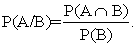(1).

In the same way one finds that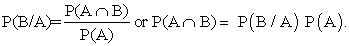Therefore (1) can be written in the form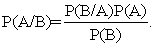(2)

Equation (2), which calculates the conditional probability P(A/B) with the help of the inverse in time conditional probability P(B/A), the prior probability P(A}and the posterior probability P(B), is known as the Bayes’ rule (or theorem, or law). In other words, the Bayes’ theorem calculates the probability of an event based on prior knowledge of conditions related to that event. When applied in practice, the Bayes’ theorem may have several interpretations. In social sciences, for example, it describes how a degree of belief expressed as a probability P(A) is rationally changed according to the availability of related evidence. In that case the probabilities involved in the Bayes’ theorem are frequently referred as Bayesian probabilities, although, mathematically speaking, Bayesian and conditional probabilities are actually the same thing.

The value of the prior probability P(A) is fixed before the experiment, whereas the value of the posterior probability is calculated with the help of the experiment’s data. Frequently, however, there exists an uncertainty about the exact value of P(A). In such cases, considering all the possible values of P(A), we obtain through the Bayes’ rule different values for the conditional probability P(A/B). Therefore, the Bayes’ rule introduces a kind of multi-valued logic tackling the existing, due to the imprecision of the value of the prior probability, uncertainty. Consequently, one could argue that Bayesian Reasoning constitutes an interface between bivalent and FL.

Although the Bayes’ rule is a straightforward consequence of equation (1) calculating the value of a conditional probability, Bayesian reasoning has been proved to be very important in everyday life situations 27, but for the whole science as well 28. Recent researches give evidence that even most of the mechanisms under which the human brain works are Bayesian 29.

Consequently, Bayesian reasoning becomes very useful for Artificial Intelligence (AI), which focuses on the design and construction of machines that mimic the human behavior. In fact, the smart machines of AI are supplied with Bayesian algorithms in order to be able to recognize the corresponding structures and to make autonomous decisions. The physicist and Nobel Prize winner John Mather has already expressed his uneasiness about the possibility that the Bayesian machines could become too smart in future, so that to make humans to look useless 30! Consequently, Sir Harold Jeffreys (1891-1989), a British mathematician who introduced the concept of the Bayesian algorithm and played an important role in the revival of the Bayesian view of probability, had successfully characterized the Bayesian rule as the “Pythagorean Theorem of Probability Theory” 31.

4.2. Examples

The Bayes rule has been proved very useful for solving problems appearing in everyday life situations. Characteristic examples of such kind of problems will be presented in this section. The first two examples are connected to the Aristotle’s fallacy of false inversion, according to which the proposition “If A then B” always implies the inverse proposition “If B then A” 12. That fallacy belongs to the category of fallacies of cause and effect, where A= the cause and B = the effect and where the cause always precedes chronically the effect.

It is of worth noting that the only information given within the premises of bivalent logic about this fallacy is that the inversion between cause and effect is false, or otherwise that the conditional probability P(A/B) is not equal to 1. However, this information is useless in practice, where one wants to know “what is” (via positiva) instead of “what is not” (via negativa). The latter, for example, is a method that has been followed by the religion when failed to define “what is the God”. It was decided then to define instead “what is not the God” (Cataphatic and Apophatic Theologies), which is much easier to be done.

Example 1: In a farm live 100 in total animals, 75 of them having 4 feet (e.g. cats, dogs, goats, cows and horses) including 3 cats and the rest of them having 2 feet (e.g. chicken). Consider the proposition. “The cats are animals having 4 feet”. Find the degree of truth of the inverse proposition “An animal living in this farm has 4 feet, therefore is a cat”.

Solution: Here we have that A=cats and B=animals having 4 feet, therefore P(B/A)=1. Consequently, equation (2) gives that P(A/B)=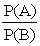. But P(A)=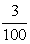, P(B)=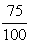, therefore P(A/B)=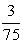= 0.04. Hence the degree of truth of the false inversion in this case is only 4%.

Nevertheless, in many cases the conditional probability P(B/A) is not equal to 1, as it happens in the following example:

Example 2: Consider the events A = I have flu and B = I feel pain in my throat. Assume that on a winter day 30% of the inhabitants of a village feel pain in their throats and that 25% of the inhabitants have flu. Assume further that the existing statistical data show that 70% of those having flu they feel pain in their throats. Find the degree of truth of the proposition “I feel pain in my throat, therefore I have flu”.

Solution: Equation (2) gives that P(A/B)=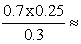0.583, or 58.3%.

Bayesian reasoning is frequently used in medical applications the outcomes of which are not always compatible to the common beliefs. The following two timely examples, due to the current COVID-19 pandemic, concern the creditability of the viruses’ diagnostic tests.

Example 3: It has been statistically shown that 2% of the inhabitants of country have been infected by a dangerous virus. Mr. X, who has not any symptoms of the corresponding disease, makes a diagnostic test, the statistical accuracy of which is 97%. The test is positive. Find the probability for Mr. X to be a carrier of the virus.

Solution: Consider the following events:

Ÿ A: The subject is a carrier of the virus.

Ÿ B: The test is positive.

From the given data it turns out that P(A)=0.02 and P(B/A)=0.97. Further, among 100 inhabitants of the country, 2 on average are carriers and 98 are no carriers of the virus. Assuming that all those people make the test, we should have on average 2x97%=1.94 positive tests from the carriers and 98x3%=2.94 positive tests from the no carriers of the virus, i.e.4.88 in total positive tests. Therefore, P(B)=0.488. Replacing the values of P(A), P(B/A) and P(B) in equation (2) one finds that P(A/B)≈0.398. Therefore, the probability for Mr. X to be a carrier of the virus is only 39.8% and not 97%, as it could be thought through a first, rough estimation!

This means that Mr. X has to make a second test to see what really happens with his health condition. Further, if the second test is negative, a third test will be also required. At the same time, however, there is an urgent need for other people to make the test. This becomes evident by the next example.

Example 4: Assume that Mr. X has some suspicious symptoms and that 85% of the people presenting such symptoms have been infected by the virus. Mr. X makes the test, which is positive. What is now the probability for Mr, X to be a carrier of the virus?

Solution: Let A and B be the events defined in Example 3. Here we have that P(A)=0.85 and P(B/A)=0.97. Further, assuming that 100 people having suspicious symptoms make the test, we should have on average 85x97%=82.45 positive tests from the carriers and 15x0.3% =0.45 from the no carriers of the virus, i.e. 82.9 in total positive tests. Therefore, P(B)=0.829. Replacing the values of P(A), P(B/A) and P(B) in equation (2) one finds that P(A/B)≈0.995. In this case, therefore, the probability for Mr. X to be a carrier of the virus is 99.5%, i.e. exceeds the statistical accuracy of the test!

In general, the sensitivity of the solution is great, depending on the values of the prior probability P(A). The greater the value of P(A), the higher the creditability of the test.

The Bayes’ rule is frequently used together with the theorem of total probability 5 for the solution of the corresponding problems. The next example illustrates this case.

Example 5: A country consists of three confederate districts, say D1, D2 and D3, where it lives 20%, 25% and 55% respectively of its total population. A percentage of 60%, 45% and 10% of the population of each one of those districts respectively is against the confederation. Find the probability for one of those people, chosen randomly, to live in district D3.

Solution: Consider the events

Ÿ Ai: A person lives in district Di, i=1, 2, 3, and

Ÿ B: A person is against the confederation

From the given data it turns out that P(A1)= 0.2, P(A2)= 0.25, P(A3)= 0.55 and P(B/A1)=0.6, P(B/A2)=0.45, P(B/A3) = 0.1. It is asked to calculate the probability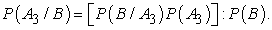(5)

The Ai’s are obviously pair wise disjoint events and their union is equal to the sample space X of the inhabitants of the country (mathematically speaking the Ai’s form a partition of X). Therefore, by the theorem of total probability 5 one finds that

P(B) = P(A1∩B)+P(A2∩B)+P(A3∩B) and by the Bayes’ rule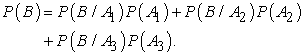(6)

Replacing the values of the probabilities involved in equation (6) one finds that P(B)=0.2875. Therefore, equation (5) gives that P(A3/B)≈0.0628 or 6.28%.

Closing this section, it is of worth making a reference to the famous accident of the Air France flight AF 447 from Rio de Janeiro to Paris on June 1, 2009, which disappeared during stormy weather over a remote part of the Atlantic carrying 228 passengers and crew to death. The aircraft’s wreckage was found two years later, only after applying Bayesian Statistics based on the data of the previous failures to find it 32.

4.3 Bayesian Reasoning in Science

Many scientists and philosophers of science argue today that the whole science could be considered as a Bayesian process 27, 28, 29. Here, we shall attempt to justify theoretically this view.

The scientific thinking process is graphically represented in Figure 4, retrieved from 28. In that figure, a1, a2,…,an are observations of the real world that have led by induction (intuitively) to the development of theory T1. Theory T1 was verified by deduction and additional deductive inferences K1, K2, …., Ks were obtained. Next, a new series of observations b1, b2,…,bm follow. If some of those observations are not compatible to the laws of theory T1, a new theory T2 is developed to replace/extend T1, and so on. In each case the new theory extends or rejects the previous one approaching more and more to the absolute truth.

This procedure is known as the scientific method. The term was introduced during the 19th century, when significant terminologies appeared establishing clear boundaries between science and not science. However, the scientific method characterizes the development of science since at least the 17th century. Aristotle (384-322 BC) is recognized as the inventor of the scientific method due to his refined analysis of the logical implications contained in demonstrative discourse. The first book in the history of human civilization written on the basis of the principles of the scientific method is, according to the existing witnesses, the “Elements” of Euclid (365-300 BC) addressing the axiomatic foundation of Geometry.

• Figure 4. The scientific method

The scientific method is highly based on the Trial and Error procedure, a term introduced by C. Lloyd Morgan (1852-1936) 33. The trial and error procedure is characterized by repeated attempts, which are continued until success or until the subject stops trying.

As an example, the geocenrtic theory (Almagest) of Ptolemy of Alexandria (100-170), being able to predict satisfactorily the movements of the planets and the moon, was considered to be true for centuries. However, it was finally proved to be wrong and has been replaced by the heliocentric theory of Copernicus (1473-1543). The Copernicus’ theory was supported and enhanced a hundred years later by the observations/studies of Kepler and Galileo, but it faced many obstacles for a long period, especially from the church, before its final justification 34. Another characteristic example is the Einstein’s general theory of relativity developed at the beginning of the 20th century. This theory has replaced the Newton’s classical gravitational theory, which was believed to be true for more than two centuries 35.

The previous discussion reveals the importance of inductive reasoning for scientific thinking. In fact, the premises of all the scientific theories (with possible exception only for pure mathematics), expressed by axioms, basic principles, etc., are based on human intuition and inductive reasoning. Therefore, a deductive inference developed on the basis of a scientific theory, is true under the CONDITION that the premises of the corresponding theory are true. In other words, if H denotes the hypothesis imposed by those premises and I denotes the deductive inference, then the conditional probability P(I/H), which can be calculated by the Bayes’ rule, expresses the degree of truth of the deductive inference. Consequently, the argument that the whole science can be considered as a Bayesian process seems to have a reasonable basis.

It must be emphasized here that the error of the inductive reasoning is transferred to a deductive inference through its premises. Therefore, the scientific error in its final form is actually a deductive and not an inductive error! This means that none of the existing scientific theories could be considered as been absolutely true; it simply could be considered as approaching in a better way the truth than the previous theories that has replaced, did.

### 5. Conclusions

The discussion performed in this paper leads to the following conclusions:

Ÿ PS is a complex cognitive process that needs the combination of several modes of thinking in order to be successful. Those modes, apart from the simple spontaneous thinking, include CrT, ST and CT.

Ÿ Probability and Statistics are related areas of mathematics, having however fundamental differences. Probability deals with predicting the likelihood of future events, whereas Statistics tries to make sense of observations in the real world by analyzing the frequency of past events.

Ÿ The cultivation of ST is very important for PS, because enables one to combine harmonically principles of Probability and Statistics for solving problems with uncertain outcomes characterized by randomness.

Ÿ Methods of FL are usually applied today for tackling the problems of uncertainty due to imprecision of the given data. Bayesian Reasoning, however, where the outcomes change by considering all the possible values of the prior probability, could be seen as an interface between bivalent and FL for tackling such kind of problems.

Ÿ Characteristic examples were presented in this work illustrating the, recently full recognized, importance of Bayesian Reasoning for everyday life and science.

### References

  Polya, G., How I solve it: A new aspect of mathematical method, New Jersey: Princeton University Press, 1973. In article  Schoenfeld, A., “The wild, wild, wild, wild world of problem solving: A review of sort”|, For the Learning of Mathematics, 3, 40-47, 1983. In article  Green, A. J. K. & Gillhooly, K., “Problem solving”, in Braisby, N. & Gelatly, A. (Eds.), Cognitive Psychology, Oxford University Press, Oxford, 2005 In article  Martinez, M., “What is metacognition? Teachers intuitively recognize the importance of metacognition, but may not be aware of its many dimensions”, Phi Delta Kappan, 87(9), 696-714, 2007. In article View Article  Schuler, J. & Lipsch utz, S., Schaum’s Outline of Probability, 2nd Edition, McGraw Hill, NY, USA, 2010. In article  Jaynes, E.T., Probability Theory: The Logic of Science, 8th Printing, Cambridge University Press, UK, 2011. In article  Mumford, D., “The Dawning of the Age of Stochasticity”, in V. Amoid, M. Atiyah, P. Laxand & B. Mazur (Eds.), Mathematics: Frontiers and Perspectives, AMS, 197-218, 2000. In article  Kosko, B., Fuzzy Thinking: The New Science of Fuzzy Logic, Hyperion, NY, USA, 1993. In article View Article  Zadeh, L.A., “Fuzzy Sets”, Information and Control, 8, 338-353, 1965. In article View Article  Voskoglou, M.Gr., “Methods for Assessing Human-Machine Performance under Fuzzy Conditions”, Mathematics, 7, article 230, 2019. In article View Article  Changingminds.org, “Full alphabetic list of Fallacies”, available online at: http://changingminds.org/disciplines/argument /fallacies/fallacies_alpha.htm (accessed on 23 June 2020) In article  Athanassopoulos, E. and Voskoglou, M.Gr., “Quantifying the Aristotle’s Fallacies”, Mathematics, 8, article 1399, 2020. In article View Article  Voskoglou, M. Gr. & Buckley, S., “Problem Solving and Computers in a Learning Environment”, Egyptian Computer Science Journal, 36(4), 28-46, 2012. In article  Halpern, D., Thought and knowledge: An introduction to critical thinking, 4th edition, Mahwah, Earlbaum, NJ, USA, 2003. In article  Warburton, N., A little history of Philosophy, Yale University Press, USA, 2011. In article  Gardener, J. & Resnik, D., “The misuse of statistics, concepts, tools and a research agenda”, Accountability in Research: Policies and Quality Assurance, 9(2), 65-74, 2002. In article View Article  PubMed  Wason, P. C., “Self-contradictions, in Johnson-Laird”, P. N.; Wason, P. C. (Eds.), Thinking: Readings in cognitive science, Cambridge, Cambridge University Press, 1977. In article  Evans, J.St.B.T., Newstead, S. E., Byrne, R.M.J., Human Reasoning: The Psychology of Deduction, Psychology Press, East Sussex, UK, 1993. In article  Tversky, A., & Kahneman, D., Judgment under uncertainty: Heuristics and biases, Cambridge University Press, Cambridge, UK, 1982. In article View Article  Aristidou, M., “Irrationality Re-Examined: A Few Comments on the Conjunction Fallacy”, Open Journal of Philosophy, 3(2), 329-336, 2013. In article View Article  Papert, S., “An exploration in the space of Mathematics Education”, International Journal of Computers for Mathematics, 1(1), 95-123., 1996. In article View Article  Wing, J. M., “Computational thinking”, Communications of the ACM, Vol.49, 33-35, 2006. In article View Article  Liu, J. & Wang, L., “Computational Thinking in Discrete Mathematics”, IEEE 2nd International Workshop on Education Technology and Computer Science, 413-416, 2010. In article View Article  PubMed  Matlin, W. M., Cognition, Wiley & Sons, New York, 2005. In article  Mc Guinness, C., “Teaching thinking: New signs for theories of cognition”, Educational Psychology, 13(3-4), 305-316, 1993. In article View Article  Kazimoglu, C., Kiernan, M., Bacon, L. & MacKinnon, L., “Understanding Computational Thinking Before Programming: Developing Guidelines for the Design of Games to Learn Introductory Programming Through Game-Play”, International Journal of Game-Based Learning, 1(3), 30-52, 2011. In article View Article  Horgan, J., “Bayes’ Theorem: What is the Big Deal?”, available at http//:blogs.scientificamerican.com/cross-check/bayes-s-theorem-what-s-the-big-deal, 2015. In article  Athanassopoulos, E. & Voskoglou, M.Gr., “A Philosophical Treatise on the Connection of Scientific Reasoning with Fuzzy Logic”, Mathematics, 8, article 875, 2020. In article View Article  Bertsch McGrayne, S., The Theory that would not die, Yale University Press, New Haven and London, 2012. In article  What do you think about machines that think?, available at http://edge.org/response-detail/26871, 2015. In article  Jeffreys, H., Scientific Inference, 3d Edition, Cambridge University Press, UK, 1973. In article  Stone, L.D., Keller, C.M., Kratzke, T.M. & Strumpfer, J.F., “Search for the Wreckage of the Air France Flight AF 447, Statistical Science, 29(1), 69-80, 2014. In article View Article  Thrope, W.H, The origins and rise of ethology: The science of the natural behavior of animals, Praeger, London-NY, 1979. In article  Gingerich, O., TheEye of the Heaven - Ptolemy, Copernicus, Kepler, American Institute of Physics, NY, USA, 1993. In article  Singh, S., “Bing Bang - The Origin of the Universe”, Harper Perennian Publishers, NY, USA, 2005. In article

Published with license by Science and Education Publishing, Copyright © 2020 Michael Gr. Voskoglou and Evangelos AthanassopoulosThis work is licensed under a Creative Commons Attribution 4.0 International License. To view a copy of this license, visit http://creativecommons.org/licenses/by/4.0/